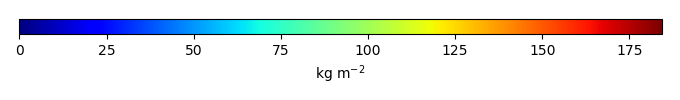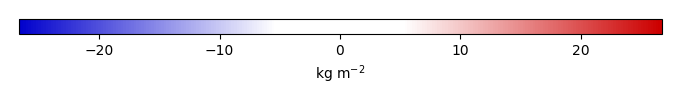# Mean State

Period Mean (original grids) [Pg]
Model Period Mean (intersection) [Pg]
Model Period Mean (complement) [Pg]
Benchmark Period Mean (intersection) [Pg]
Benchmark Period Mean (complement) [Pg]
Bias [kg m-2]
Bias Score 
Spatial Distribution Score 
Overall Score 
Benchmark [-] 34.1
CLM4 [-] 31.5 32.8 0.148 33.8 0.315 0.0325 0.647 0.891 0.769
CLM4.5 [-] 38.1 38.5 0.300 33.8 0.315 1.53 0.596 0.772 0.684
CLM5 [-] 41.4 42.1 0.279 33.8 0.315 2.30 0.618 0.799 0.708
Period Mean (original grids) [Pg]
Model Period Mean (intersection) [Pg]
Model Period Mean (complement) [Pg]
Benchmark Period Mean (intersection) [Pg]
Benchmark Period Mean (complement) [Pg]
Bias [kg m-2]
Bias Score 
Spatial Distribution Score 
Overall Score 
Benchmark [-] 44.8
CLM4 [-] 22.1 20.8 0.00819 44.4 0.478 -3.43 0.591 0.887 0.739
CLM4.5 [-] 17.6 16.7 0.0228 44.4 0.478 -4.03 0.534 0.762 0.648
CLM5 [-] 17.5 16.5 0.0170 44.4 0.478 -4.09 0.509 0.847 0.678
Period Mean (original grids) [Pg]
Model Period Mean (intersection) [Pg]
Model Period Mean (complement) [Pg]
Benchmark Period Mean (intersection) [Pg]
Benchmark Period Mean (complement) [Pg]
Bias [kg m-2]
Bias Score 
Spatial Distribution Score 
Overall Score 
Benchmark [-] 60.1
CLM4 [-] 27.6 27.0 0.389 59.3 0.762 -3.26 0.576 0.831 0.704
CLM4.5 [-] 36.0 34.9 0.936 59.3 0.762 -2.13 0.627 0.898 0.762
CLM5 [-] 40.1 38.8 0.989 59.3 0.762 -1.70 0.587 0.785 0.686
Period Mean (original grids) [Pg]
Model Period Mean (intersection) [Pg]
Model Period Mean (complement) [Pg]
Benchmark Period Mean (intersection) [Pg]
Benchmark Period Mean (complement) [Pg]
Bias [kg m-2]
Bias Score 
Spatial Distribution Score 
Overall Score 
Benchmark [-] 11.1
CLM4 [-] 7.93 0.889 7.17 10.3 0.803 -16.4 0.430 0.242 0.336
CLM4.5 [-] 93.5 8.35 85.4 10.3 0.803 7.44 0.444 0.208 0.326
CLM5 [-] 248. 14.7 234. 10.3 0.803 23.0 0.397 0.0745 0.236
Period Mean (original grids) [Pg]
Model Period Mean (intersection) [Pg]
Model Period Mean (complement) [Pg]
Benchmark Period Mean (intersection) [Pg]
Benchmark Period Mean (complement) [Pg]
Bias [kg m-2]
Bias Score 
Spatial Distribution Score 
Overall Score 
Benchmark [-] 55.7
CLM4 [-] 24.7 23.3 1.21 50.7 4.99 -5.18 0.682 0.184 0.433
CLM4.5 [-] 29.3 27.4 1.64 50.7 4.99 -3.00 0.690 0.360 0.525
CLM5 [-] 25.3 23.5 1.53 50.7 4.99 -4.37 0.693 0.301 0.497
Period Mean (original grids) [Pg]
Model Period Mean (intersection) [Pg]
Model Period Mean (complement) [Pg]
Benchmark Period Mean (intersection) [Pg]
Benchmark Period Mean (complement) [Pg]
Bias [kg m-2]
Bias Score 
Spatial Distribution Score 
Overall Score 
Benchmark [-] 65.6
CLM4 [-] 85.6 37.9 45.7 65.2 0.461 -6.33 0.618 0.470 0.544
CLM4.5 [-] 156. 67.7 84.9 65.2 0.461 1.04 0.671 0.832 0.752
CLM5 [-] 294. 93.5 195. 65.2 0.461 7.25 0.621 0.942 0.781
Period Mean (original grids) [Pg]
Model Period Mean (intersection) [Pg]
Model Period Mean (complement) [Pg]
Benchmark Period Mean (intersection) [Pg]
Benchmark Period Mean (complement) [Pg]
Bias [kg m-2]
Bias Score 
Spatial Distribution Score 
Overall Score 
Benchmark [-] 143.
CLM4 [-] 86.3 84.2 4.13 141. 2.09 -2.99 0.591 0.848 0.719
CLM4.5 [-] 118. 114. 7.42 141. 2.09 -1.12 0.610 0.743 0.677
CLM5 [-] 122. 112. 14.0 141. 2.09 -1.29 0.660 0.741 0.700
Period Mean (original grids) [Pg]
Model Period Mean (intersection) [Pg]
Model Period Mean (complement) [Pg]
Benchmark Period Mean (intersection) [Pg]
Benchmark Period Mean (complement) [Pg]
Bias [kg m-2]
Bias Score 
Spatial Distribution Score 
Overall Score 
Benchmark [-] 58.4
CLM4 [-] 36.4 34.9 0.544 56.8 1.57 -2.35 0.653 0.817 0.735
CLM4.5 [-] 46.4 44.4 1.04 56.8 1.57 -0.617 0.640 0.667 0.653
CLM5 [-] 37.4 35.7 0.948 56.8 1.57 -1.91 0.633 0.766 0.700
Period Mean (original grids) [Pg]
Model Period Mean (intersection) [Pg]
Model Period Mean (complement) [Pg]
Benchmark Period Mean (intersection) [Pg]
Benchmark Period Mean (complement) [Pg]
Bias [kg m-2]
Bias Score 
Spatial Distribution Score 
Overall Score 
Benchmark [-] 7.78
CLM4 [-] 5.53 0.647 5.33 7.36 0.414 -13.9 0.425 0.239 0.332
CLM4.5 [-] 95.5 8.25 88.6 7.36 0.414 9.15 0.428 0.256 0.342
CLM5 [-] 254. 13.8 243. 7.36 0.414 26.1 0.437 0.0664 0.252
Period Mean (original grids) [Pg]
Model Period Mean (intersection) [Pg]
Model Period Mean (complement) [Pg]
Benchmark Period Mean (intersection) [Pg]
Benchmark Period Mean (complement) [Pg]
Bias [kg m-2]
Bias Score 
Spatial Distribution Score 
Overall Score 
Benchmark [-] 58.9
CLM4 [-] 15.7 15.0 0.635 57.4 1.53 -4.50 0.513 0.806 0.660
CLM4.5 [-] 24.8 23.4 1.25 57.4 1.53 -3.27 0.570 0.693 0.632
CLM5 [-] 30.2 28.1 1.77 57.4 1.53 -2.42 0.531 0.377 0.454
Period Mean (original grids) [Pg]
Model Period Mean (intersection) [Pg]
Model Period Mean (complement) [Pg]
Benchmark Period Mean (intersection) [Pg]
Benchmark Period Mean (complement) [Pg]
Bias [kg m-2]
Bias Score 
Spatial Distribution Score 
Overall Score 
Benchmark [-] 61.8
CLM4 [-] 24.8 23.4 1.03 60.2 1.58 -5.97 0.592 0.406 0.499
CLM4.5 [-] 57.3 52.7 3.82 60.2 1.58 0.195 0.666 0.793 0.729
CLM5 [-] 67.9 60.9 5.98 60.2 1.58 2.18 0.700 0.954 0.827
Period Mean (original grids) [Pg]
Model Period Mean (intersection) [Pg]
Model Period Mean (complement) [Pg]
Benchmark Period Mean (intersection) [Pg]
Benchmark Period Mean (complement) [Pg]
Bias [kg m-2]
Bias Score 
Spatial Distribution Score 
Overall Score 
Benchmark [-] 1.09e+03
CLM4 [-] 614. 506. 108. 1.03e+03 55.6 -3.97 0.595 0.744 0.670
CLM4.5 [-] 1.27e+03 736. 535. 1.03e+03 55.6 -1.63 0.615 0.759 0.687
CLM5 [-] 1.92e+03 821. 1.10e+03 1.03e+03 55.6 -0.830 0.621 0.437 0.529
Period Mean (original grids) [Pg]
Model Period Mean (intersection) [Pg]
Model Period Mean (complement) [Pg]
Benchmark Period Mean (intersection) [Pg]
Benchmark Period Mean (complement) [Pg]
Bias [kg m-2]
Bias Score 
Spatial Distribution Score 
Overall Score 
Benchmark [-] 64.3
CLM4 [-] 5.51 5.39 0.102 63.6 0.726 -3.53 0.413 0.576 0.494
CLM4.5 [-] 11.5 11.3 0.279 63.6 0.726 -3.10 0.462 0.796 0.629
CLM5 [-] 18.3 18.0 0.405 63.6 0.726 -2.65 0.500 0.760 0.630
Period Mean (original grids) [Pg]
Model Period Mean (intersection) [Pg]
Model Period Mean (complement) [Pg]
Benchmark Period Mean (intersection) [Pg]
Benchmark Period Mean (complement) [Pg]
Bias [kg m-2]
Bias Score 
Spatial Distribution Score 
Overall Score 
Benchmark [-] 13.3
CLM4 [-] 23.0 1.32 21.5 12.1 1.24 -8.66 0.433 0.303 0.368
CLM4.5 [-] 126. 8.66 117. 12.1 1.24 0.497 0.438 0.531 0.484
CLM5 [-] 326. 17.3 310. 12.1 1.24 10.0 0.425 0.140 0.283
Period Mean (original grids) [Pg]
Model Period Mean (intersection) [Pg]
Model Period Mean (complement) [Pg]
Benchmark Period Mean (intersection) [Pg]
Benchmark Period Mean (complement) [Pg]
Bias [kg m-2]
Bias Score 
Spatial Distribution Score 
Overall Score 
Benchmark [-] 42.5
CLM4 [-] 7.50 7.00 0.290 40.2 2.26 -9.53 0.473 0.280 0.376
CLM4.5 [-] 21.0 19.4 1.02 40.2 2.26 -3.94 0.560 0.814 0.687
CLM5 [-] 21.0 19.3 1.14 40.2 2.26 -3.97 0.574 0.835 0.704
Period Mean (original grids) [Pg]
Model Period Mean (intersection) [Pg]
Model Period Mean (complement) [Pg]
Benchmark Period Mean (intersection) [Pg]
Benchmark Period Mean (complement) [Pg]
Bias [kg m-2]
Bias Score 
Spatial Distribution Score 
Overall Score 
Benchmark [-] 45.3
CLM4 [-] 33.2 32.4 0.299 44.4 0.888 -2.50 0.693 0.579 0.636
CLM4.5 [-] 53.3 52.0 0.511 44.4 0.888 2.52 0.627 0.908 0.768
CLM5 [-] 42.8 41.7 0.410 44.4 0.888 -0.109 0.748 0.836 0.792
Period Mean (original grids) [Pg]
Model Period Mean (intersection) [Pg]
Model Period Mean (complement) [Pg]
Benchmark Period Mean (intersection) [Pg]
Benchmark Period Mean (complement) [Pg]
Bias [kg m-2]
Bias Score 
Spatial Distribution Score 
Overall Score 
Benchmark [-] 51.3
CLM4 [-] 41.7 25.6 16.3 49.6 1.76 -6.92 0.626 0.633 0.629
CLM4.5 [-] 75.3 42.8 33.0 49.6 1.76 -1.38 0.670 0.884 0.777
CLM5 [-] 145. 61.9 81.7 49.6 1.76 4.53 0.624 0.721 0.672
Period Mean (original grids) [Pg]
Model Period Mean (intersection) [Pg]
Model Period Mean (complement) [Pg]
Benchmark Period Mean (intersection) [Pg]
Benchmark Period Mean (complement) [Pg]
Bias [kg m-2]
Bias Score 
Spatial Distribution Score 
Overall Score 
Benchmark [-] 34.3
CLM4 [-] 11.8 11.9 0.338 34.0 0.216 -5.52 0.538 0.790 0.664
CLM4.5 [-] 14.5 14.9 0.385 34.0 0.216 -4.64 0.590 0.904 0.747
CLM5 [-] 12.9 13.1 0.522 34.0 0.216 -5.04 0.549 0.830 0.689
Period Mean (original grids) [Pg]
Model Period Mean (intersection) [Pg]
Model Period Mean (complement) [Pg]
Benchmark Period Mean (intersection) [Pg]
Benchmark Period Mean (complement) [Pg]
Bias [kg m-2]
Bias Score 
Spatial Distribution Score 
Overall Score 
Benchmark [-] 101.
CLM4 [-] 77.2 76.5 0.209 100. 0.811 -2.53 0.726 0.383 0.554
CLM4.5 [-] 90.8 90.1 0.454 100. 0.811 -0.578 0.714 0.677 0.695
CLM5 [-] 86.4 85.4 0.425 100. 0.811 -1.19 0.725 0.587 0.656
Period Mean (original grids) [Pg]
Model Period Mean (intersection) [Pg]
Model Period Mean (complement) [Pg]
Benchmark Period Mean (intersection) [Pg]
Benchmark Period Mean (complement) [Pg]
Bias [kg m-2]
Bias Score 
Spatial Distribution Score 
Overall Score 
Benchmark [-] 60.7
CLM4 [-] 37.5 37.7 0.238 60.4 0.300 -3.03 0.668 0.724 0.696
CLM4.5 [-] 48.2 48.0 0.511 60.4 0.300 -1.23 0.651 0.885 0.768
CLM5 [-] 62.6 63.0 0.653 60.4 0.300 1.03 0.652 0.913 0.783
Period Mean (original grids) [Pg]
Model Period Mean (intersection) [Pg]
Model Period Mean (complement) [Pg]
Benchmark Period Mean (intersection) [Pg]
Benchmark Period Mean (complement) [Pg]
Bias [kg m-2]
Bias Score 
Spatial Distribution Score 
Overall Score 
Benchmark [-] 29.6
CLM4 [-] 6.54 6.40 0.120 28.2 1.46 -6.16 0.487 0.290 0.389
CLM4.5 [-] 10.7 10.4 0.165 28.2 1.46 -4.64 0.537 0.561 0.549
CLM5 [-] 17.7 16.8 0.278 28.2 1.46 -2.29 0.558 0.730 0.644

# Temporally integrated period mean

BENCHMARK MEANMODEL MEANBIASBIAS SCORESPATIAL TAYLOR DIAGRAMMODEL COLORS# Spatially integrated regional mean

MODEL COLORS# All Models

BenchmarkCLM4CLM4.5CLM5# Data Information

Approach: I just read the data from the original data file and flip the latitude arrange from original 90N->90S to 90S->90N.

Temporal resolution: yearly

General information: This product was derived from Harmonized World Soil Database (HWSD) v1.2 dataset.

Spatial resolution: 0.5x0.5 degree